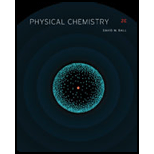# Determine under what conditions of temperature and wavelength the Rayleigh-Jeans law approximates Planck’s law.### Physical Chemistry

2nd Edition
Ball + 3 others
ISBN: 9781133958437### Physical Chemistry

2nd Edition
Ball + 3 others
ISBN: 9781133958437

#### Solutions

Chapter
Section
Chapter 9, Problem 9.59E
Textbook Problem
54 views

## Determine under what conditions of temperature and wavelength the Rayleigh-Jeans law approximates Planck’s law.

Expert Solution
Interpretation Introduction

Interpretation:

The conditions of temperature and wavelength at which the Rayleigh-Jeans law approximates Planck’s law are to be calculated.

Concept introduction:

Planck’s equation can also be represented in the form of energy density distribution of a black body radiation at a given temperature and wavelength. This equation is known as Planck’s radiation distribution law.

Rayleigh-Jeans law gives the relation between infinitesimal amount of energy per unit volume and wavelength interval for a blackbody radiation. The relation is shown below.

dρ=8πkTλ4dλ

### Explanation of Solution

The Planck’s law is given by,

dρ=8πhcλ5(1ehc/λkT1)dλ(1)

The Taylor series for the exponential term is,

ex=1+x+x22!+.........

In this case, the value of x=hcλkT

Apply first order approximation to the Taylor series of exponential for the Planck’s law as shown below.

ehcλkT1+hcλkT(2)

Substitute the value of exponential given in equation (2) in the equation (1)

### Want to see the full answer?

Check out a sample textbook solution.See solution

### Want to see this answer and more?

Bartleby provides explanations to thousands of textbook problems written by our experts, many with advanced degrees!

See solution